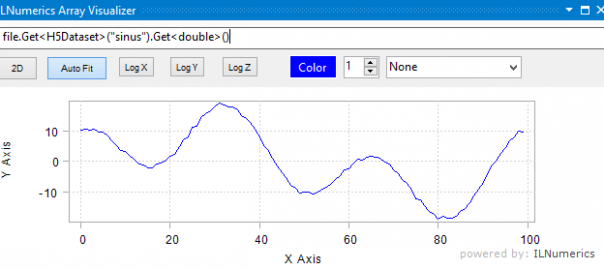# HDF5 and Matlab Files – Fun with ILNumerics

### Why to use HDF5 and ILNumerics?

HDF5 is a file format (Hierarchical Data Format) especially desgined to handle huge amount of numerical data. Just to mention an example,  NASA chose it to be the standard file format for storing data from the Earth Observing System (EOS).

ILNumerics easily handles HDF5 files. They can be used to exchange data with other software tools, for example Matlab mat files. In this post I will show a step by step guide – how to interface ILNumerics with Matlab.

### Save Matlab data to HDF5 format

Let’s start by creating some arbitrary data with Matlab and saving it in HDF5 format, Matlab supports it from 2006 September, version 7.3.

matrix = randn(10, 10) * 5;
vector = [1 2 3 4 5]';
carr = 'It is a char array!';
noise = rand(1,100) - rand(1, 100);
sinus = 10 * sin(linspace(0, 2*pi, 100)) + noise;
complex = rand(1, 5) * (10 + 10i);
save('test_hdf5.mat', '-v7.3');


### Load Matlab data with ILNumerics

Let’s now see what ILNumerics sees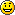Matlab simply stored all arrays as individual datasets in the root node of the HDF5 file. It is now easily possible to access the data! We could take a peek and inspect them right within the Visual Studio debugger visualizers. But let’s see how the data is loaded programmatically:

// Reading from Matlab
using (H5File file = new H5File("test_hdf5.mat"))
{
using (ILScope.Enter())
{
ILArray<double> matrix = file.Get<H5Dataset>("matrix").Get<double>();
ILArray<double> vector = file.Get<H5Dataset>("vector").Get<double>();
ILArray<char> carr = file.Get<H5Dataset>("carr").Get<char>();
ILArray<double> noise = file.Get<H5Dataset>("noise").Get<double>();
ILArray<double> sinus = file.Get<H5Dataset>("sinus").Get<double>();
}
}



The datasets are loaded – and the whole process was very easy. It took me less than ten minutes to figure out the methods. It would have been even less if I had take a look on the examples of ILNumericsNow, let’s startup the Array Visualizer! Just write ‘array’ in the Quick Launch box of Visual Studio, or open a new visualizer window via VIEW -> OTHER WINDOWS -> Array Visualizer. In the visualizer expression field enter: ‘sinus’. The array is now visualized, how cool is that### Visualizing Matlab mat files – on the fly

Now we have shown the content of the variable ‘sinus’. Just as well, we could visualize the content of the HDF5 file and have the visualizer follow any changes to it immediately: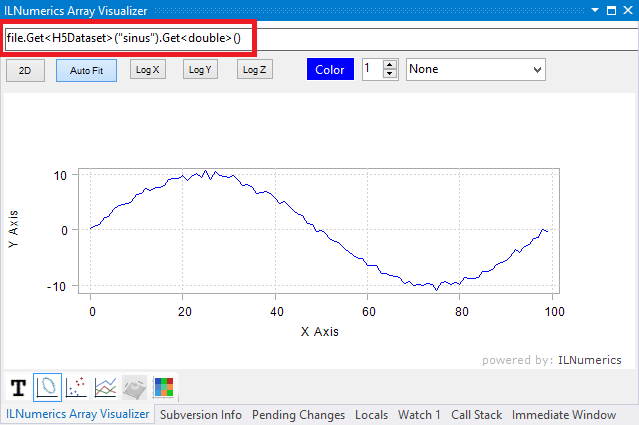If we multiply it with -1, the expression is immediately evaluated: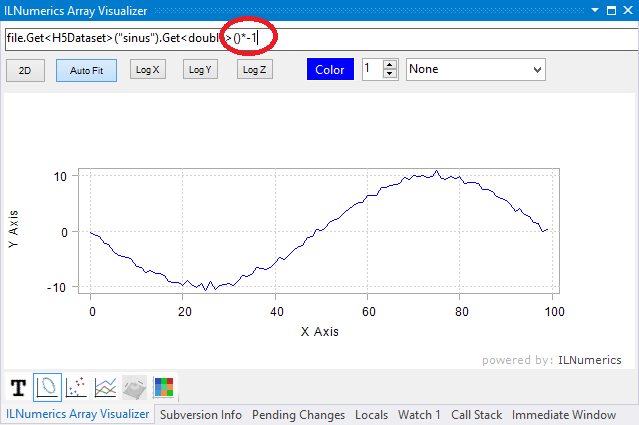And what is even more interesting, if we change the HDF5 file interactively, in the immediate window, the ILNumerics Array Visualizer reflects that on the fly:

Now let us check, what happens if we want to go from ILNumerics to Matlab. Saving a noise cosine with the following code:

// Writing some data to HDF5
// Note: It will not make it a proper mat file, only HDF5!
if (File.Exists("test_iln.mat"))
File.Delete("test_iln.mat");

using (H5File newFile = new H5File("test_iln.mat"))
{
using (ILScope.Enter())
{
ILArray<double> noisyCos = ILMath.cos(ILMath.linspace(0, 3 * Math.PI, 1000)) * 10 + ILMath.rand(1, 1000);
H5Dataset set = new H5Dataset("noisyCos", noisyCos);
}
}


Since the ILNumerics HDF5 is not a mat file format, the only way to load it (as for now) is with one of the ‘h5*’ methods of Matlab. To check the info and load, the following code is used:

h5info('test_iln.mat', '/')


In this way, it is possible to load ILArrays into Matlab### Altering Matlab mat files in ILNumerics

It is possible to alter loaded mat files with some limitations. What I found is that the length of the array can not be changed. Obviously, Matlab mat files are created as datasets without chunks, so the dataspace size cannot be changed after the file was created. However, one can alter the data (without changing the size) :

// Altering data of mat file (v7.3 format)
using (H5File file = new H5File("test_hdf5.mat"))
{
using (ILScope.Enter())
{
ILArray<double> sinus = file.Get<H5Dataset>("sinus").Get<double>();
sinus.a = sinus * -1;
file.Get<H5Dataset>("sinus").Set(sinus, "0;:");
}
}


Let me draw your attention to the use of the Set function here. I first tried to use:

file.Get<H5Dataset>("dsname").Set(A);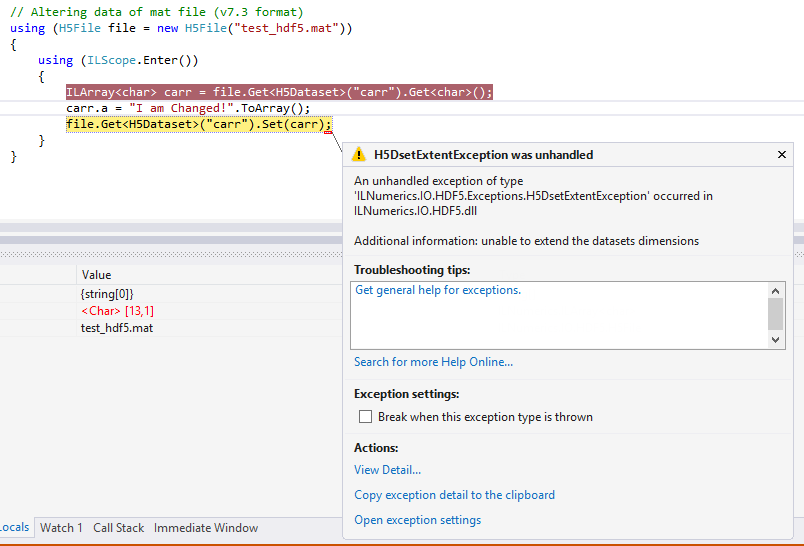But this would attempt to overwrite the whole dataset – something which could potentially change the size, hence would require a chunked dataset. Therefore, I figured that I have to be more specific and need to provide the target data range also:

file.Get<H5Dataset>("sinus").Set(sinus, "0;:")


### More Limitations

Out of curiosity I checked the complex array, to see if it is okay.